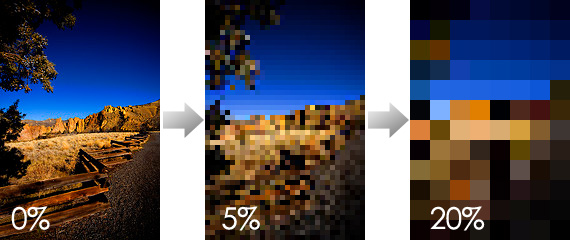# GD

## Simple Image Resize Calculator Function

Here is a simple function to calculate the width and height of an image given the constraints you want the image to fit in. This is great for creating thumbnails which need to fit into a specific height / width.

To call the function, pass in the current height / width of the image you’d like to resize along with the constrain dimensions of the final product. In this case, I’d like the thumbnail to fit within the constraints 125px X 125px. So, no matter what the dimensions returned will be within those confines.

If you don’t pass along a `\$constraintWidth` or `\$constraintHeight` [or pass them as `0`], they will be set to the `\$width` / `\$height`.

Also, all parameters are assumed to be of the strict type `int`.

``````
<?php
function getImageResizeDimensions(\$width,\$height,\$constraintWidth = 0,\$constraintHeight = 0) {
\$constraintWidth = (\$constraintWidth === 0) ? \$width : \$constraintWidth;
\$constraintHeight = (\$constraintHeight === 0) ? \$height : \$constraintHeight;

if ((\$width > \$constraintWidth) || (\$height > \$constraintHeight)) {
while ((\$constraintWidth < \$width) || (\$constraintHeight < \$height)) {
if (\$constraintWidth < \$width) {
\$height = intval(floor(((\$constraintWidth * \$height) / \$width)));
\$width = \$constraintWidth;
}
if (\$constraintHeight < \$height) {
\$width = intval(floor(((\$constraintHeight * \$width) / \$height)));
\$height = \$constraintHeight;
}
}
}

# Some super short || skinny images will return 0 for these. Make sure that doesn't happen!
if (\$width < 1) {
\$width = 1;
}
if (\$height < 1) {
\$height = 1;
}

return array (\$width,\$height);
}

\$imageURI = "/path/to/image.jpg";
list (\$width,\$height) = getimagesize(\$imageURI);
list (\$newWidth,\$newHeight) = getImageResizeDimensions(\$width,\$height,125,125);

echo "newWidth: \$newWidth\n";
echo "newHeight: \$newHeight\n";
?>

``````
Read Full Post | Make a Comment ( 1 so far )

## Creating Pixelated / Mosaic images with GD in PHP

A simple way to create a pixelated [or mosaic-looking] image in PHP using GD is to, in theory:

1. Create a image resource for the soon-to-be-pixelated image
2. Resize the image to 5% of it’s original size [adjust to taste]
3. Save image to server or send to browser

Using the script below will turn the image on the left into the images on the right …Photo by pinklimoncello.

The left-most image is at 0% pixelation [and is the original image]. The middle middle image is at 5%, and right right-most image is at 20% pixelation.

Have at it!

``````
<?php
\$img = imagecreatefromjpeg("image.jpg");
\$width = imagesx(\$img);
\$height = imagesy(\$img);

# Create 5% version of the original image:
\$newImg = imagecreatetruecolor(\$width,\$height);
imagecopyresized(\$newImg,\$img,0,0,0,0,round(\$width / 5),round(\$height / 5),\$width,\$height);

# Create 100% version ... blow it back up to it's initial size:
\$newImg2 = imagecreatetruecolor(\$width,\$height);
imagecopyresized(\$newImg2,\$newImg,0,0,0,0,\$width,\$height,round(\$width / 5),round(\$height / 5));

# No need for a jpeg here :-)
imagepng(\$newImg2,"imagePixelated.png");
?>

``````
Read Full Post | Make a Comment ( None so far )